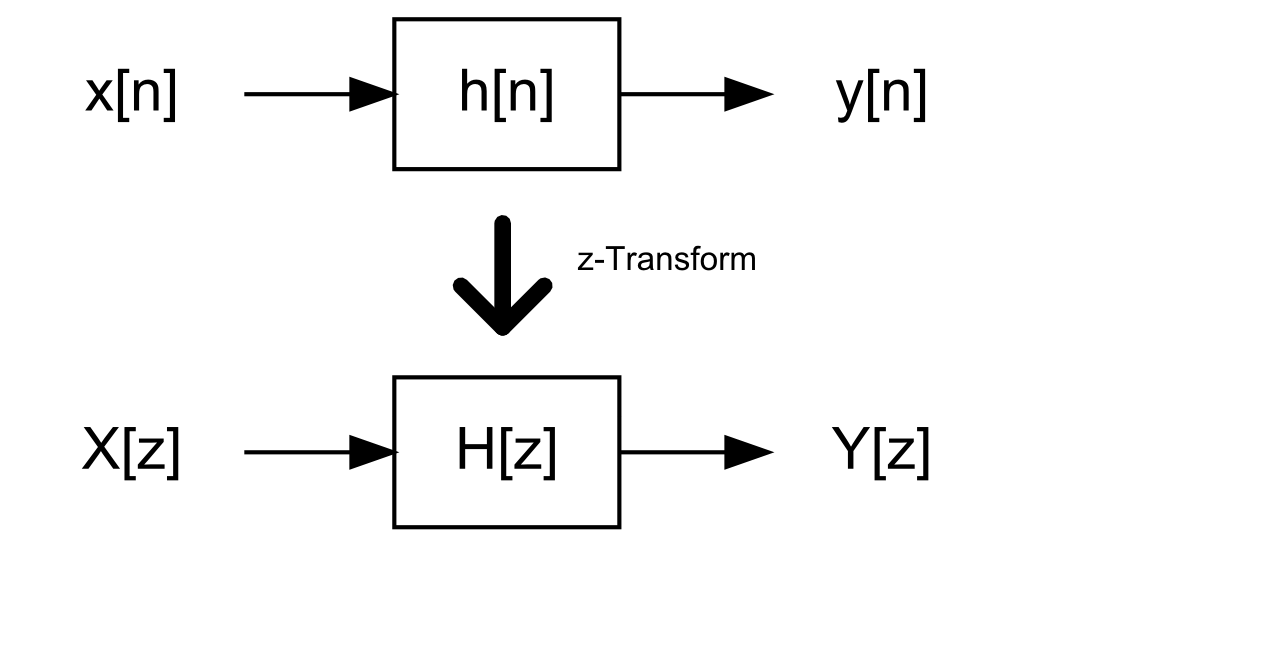# Impulse Invariant Transform

## Introduction

The impulse invariant transform (IIT) is a method of taking a continuous-time system H(s) and converting it to a discrete-time system. There are multiple ways of doing this, but the IIT does so with the constraint that the impulse response of the discrete-time system is a sampled version of the impulse response of the continuous-time system.

Here’s an illustration:…gets converted to……with the characteristic that the discrete-time impulse response is a sampled version of the continuous-time:## Rational Systems

This doesn’t seem like a big deal—nor very accurate. The illustration above implies that we’re taking an IIR response from the continuous-time system and sampling as a discrete-time FIR.

However, the IIT actually does something better: if H(s) is rational (composed of a numerator and denominator):

`H(s) = frac{N_c(s)}{D_c(s)}`

…then the IIT lets us re-write this system as a discrete-time rational system:

`H[z] = frac{N_d[z]}{D_d[z]}`

…of course, with the property that `h[n] = T_s times h(nT_s)`. `T_s` is the sampling period (typically, during transformation from continous-time to discrete-time, the continuous-time impulse response is scaled by the sample period).

## Example

Here’s an example:

`H(s) = frac{s}{s^2 + 1}`

First, we break it down into its partial fraction expansion:

`H(s) = frac{s}{(s-j)(s+j)} = frac{a}{s-j} + frac{b}{s+j}`

Solving (taking limits of s to +j and -j):

`a = frac{1}{2}`
`b = frac{-1}{2}`

Now, the IIT prescribes how to take single-pole transfer functions and convert them to the z-domain.

`frac{a}{s-j} rArr frac{T_s a}{1-e^{+jT_s}z^{-1}}`
`frac{b}{s+j} rArr frac{T_s b}{1-e^{-jT_s}z^{-1}}`

…which means that:

`H[z] = frac{T_s}{2(1-e^{+jT_s}z^{-1})} - frac{T_s}{2(1-e^{-jT_s}z^{-1})}`
`H[z] = frac{T_s cos(jT_s) z^{-1}}{1 - cos(jT_s)z^{-1} + z^{-2}}`

## Application

The impulse invariant transform is useful in modelling continuous-time sigma-delta, allowing one to analyze the mixed-mode continuous-time sigma-delta as a purely discrete-time system.

Most notably, in considering the stability of continuous-time sigma-delta ADC’s, the transformation is useful in allowing one to replace the continuous-time noise-shaping filter with a discrete-time equivalent. One could then perform a closed-loop analytic analysis on the system. This is the procedure advocated in Delta-Sigma Data Converters: Theory, Design, and Simulation. However, the book only prescribes a rule-of-thumb, and in general, one must simulate the sigma-delta rigorously to gain confidence of stability.

Arguably, this transformation was more useful in the past, when high-level mixed-mode simulators (Simulink) were not available. In that case, the only way to simulate the continous-time sigma-delta was to model at a discrete-time. Nowadays (in my experience) Simulink is fast enough that it’s easier to keep the mixed-mode nature of the system intact (i.e. not model it as a purely discrete-time system). However, I can imagine a case of either very long simulations or a regression analysis system where the cycle-accurate discrete-time model may become useful again.